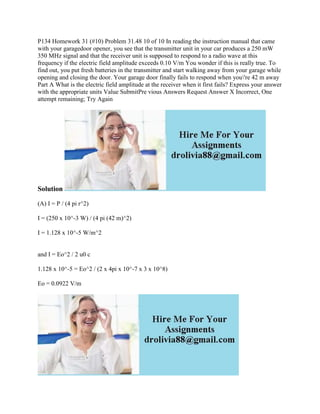Successfully reported this slideshow.

# P134 Homework 31 (#10) Problem 31-48 10 of 10 In reading the instructi.docx×

# P134 Homework 31 (#10) Problem 31-48 10 of 10 In reading the instructi.docx

Solution
(A) I = P / (4 pi r^2)
I = (250 x 10^-3 W) / (4 pi (42 m)^2)
I = 1.128 x 10^-5 W/m^2
and I = Eo^2 / 2 u0 c
1.128 x 10^-5 = Eo^2 / (2 x 4pi x 10^-7 x 3 x 10^8)
Eo = 0.0922 V/m
.

Solution
(A) I = P / (4 pi r^2)
I = (250 x 10^-3 W) / (4 pi (42 m)^2)
I = 1.128 x 10^-5 W/m^2
and I = Eo^2 / 2 u0 c
1.128 x 10^-5 = Eo^2 / (2 x 4pi x 10^-7 x 3 x 10^8)
Eo = 0.0922 V/m
.